# 前言# 代码实现

• 求出他是几位数
• 分别输出每一位数
• 按照逆序输出各位数字，比如原数字是 12345，新的数字是 54321

``````const { writeFileSync } = require('fs');
const { join } = require('path');

const generateFunction = () => {
let currentNum = 0;
let str = '';
while( currentNum < 100000) {
const stringCurrentNum = String(currentNum);
const numArr = stringCurrentNum.split('').reverse();
const unitList = ['个', '十', '百', '千', '万']
str += `    case \${currentNum}: \n`
str += `      console.log('是 \${stringCurrentNum.length} 位数');\n`;
numArr.forEach((char, index) => {
str += `      console.log('\${unitList[index]}位数是：\${char}')\n`;
})
str += `      console.log('倒过来是：\${Number(numArr.join(''))}');\n`;
str += `      break;\n`;
currentNum ++;
}
writeFileSync(join(__dirname, 'func.js'), `
function handleNumber(num) {
switch (num) {
\${str}
}
}`, {
encoding: 'utf-8'
})
}

generateFunction();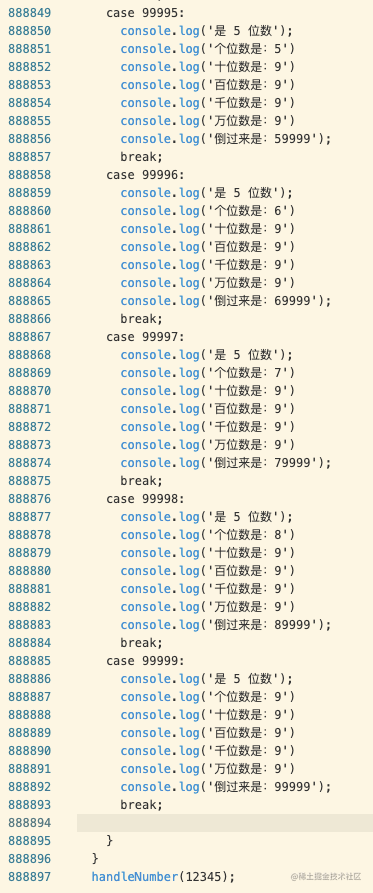88 w 行代码，穷举法，永远的神！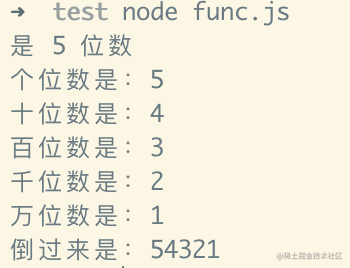# 浅析算法思想## 穷举法

• 一是问题的简化，尽可能对需要处理的问题进行模型结构上的精简。这种精简具体可体现在问题中的变量数目，减少变量的数据，从而能够从根本上降低「可能解」的组合。
• 二是对筛选「可能解」的范围和条件进行严格判断，尽可能的剔除大部分无效的「可能解」。

## 递推法

• 顺推法：从已知条件出发，逐步推算出要解决问题的方法。例如斐波那契数列就可以通过顺推法不断递推算出新的数据。
• 逆推法：从已知的结果出发，用迭代表达式逐步推算出问题开始的条件，即顺推法的逆过程。

1. 因为三角形内角和是 180 度，且其中两个角分别为 65 度和 70 度。
2. 所以第三个角是 45 度。
3. 因为平角是 180 度。
4. 所以该角的外角是 135 度。

## 分治法

• 分解，将要解决的问题划分成若干个规模较小的同类问题。
• 求解，当子问题划分得足够小时，用较简单的方法解决。
• 合并，按原问题的要求，将子问题的解逐层合并构成原问题的解。

## 贪心算法

• 不能保证最后的解是最优的。
• 不能用来求最大或最小解问题。
• 只能求满足某些约束条件的可行解的范围。

• 建立数学模型来描述问题。
• 把求解的问题分成若干个子问题。
• 对每一子问题求解，得到子问题的局部最优解。
• 把子问题的局部最优解合并成原来解问题的一个解。

## 试探法

• 针对所给问题，定义问题的解空间。
• 确定易于搜索的解空间结构。
• 以深度优先方式搜索解空间，并在搜索过程中用剪枝函数避免无效搜索。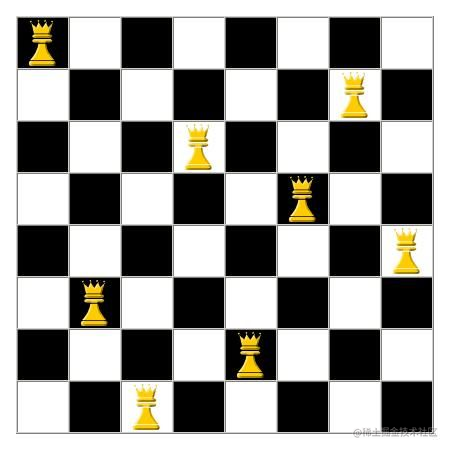## 模拟法

### 模拟法实践 ——— 求圆周率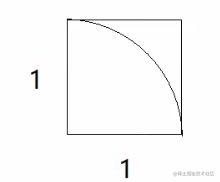``````let i = 0;
let num = 0;
const sum = 100000000;
while(i < sum) {
i ++;
const x = Math.random();
const y = Math.random();
if(x * x + y * y < 1) {
num++;
}
}
console.log(4 * num / sum);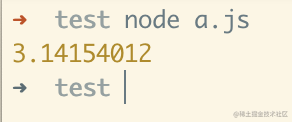# 结束语`写在最后`